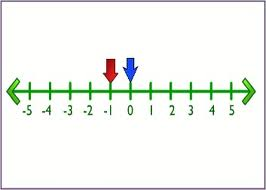Difference between Integer and Number

Key Difference: An integer refers to a whole number meaning that it is not in the form of a fraction. Integers comprise of whole numbers as well as their opposites. A number can be described as a mathematical value that is represented through a word, symbol or figure. These numbers are used to denote a particular quantity. Numbers are generally used for measuring, labeling and ordering.Integers can be described as whole numbers meaning that they do not have any fractional parts. Integers also include negative numbers. It is important to mention that 0 is also included in the list of integers. Zero is considered to be neutral which means that it is neither negative nor positive. If a number is written as a decimal or a fraction: for example, –8.00 and 12/2, both would still be referred to as integers, because they are equal to –8 and 6, respectively. Number theory is a branch of pure mathematics that is devoted primarily to the study of integers.Numbers form the base of Mathematics. The history of numbers can be traced back to the early civilizations like the one in Sumeria, around 4000 BC. Earlier, counting was done with the help of tokens. Each token used to represent a tangible entity. Clay tablets evolved in order to write the marks corresponding to the tokens. Later, Egyptians changed the concept of number; as a unit of measuring things instead of just a unit for counting. Pythagoras of Greece introduced the idea of even and odd numbers. Thus, the numbers kept on evolving and finally took a shape of an object that can be used to count, label or to measure something. A notational symbol that represents a number is known as a numeral. Numbers are generally classified according to their type. A number does not hold any importance and it only becomes significant after being associated to a group of objects with some shared characteristics.

Comparison between Integer and Number:

 Integer Number Definition An integer refers to a whole number meaning that it is not in a form of a fraction. Integers comprises of whole numbers as well as their opposites. A number can be described as a mathematical object that is used for counting. Types Positive integers- they are also know as non-negative integers.  (3,5,90) Negative integers - they are opposite of positive integers and have a prefixed sign of ‘-’. (-3, -5, -90) Natural numbers – count the elements of a whole, cardinal number or express the order which occupies an element. N= {0, 1, 2, 3, 4, 5, 6, 7, 8, 9,...} Integers - an integer refers to a whole number meaning that it is not in a form of a fraction.  (3,5,90),  (-3, -5, -90) Rational Numbers - are the quotient terms of two integers with single non-zero denominators. 3/2, -6/7. Irrational Numbers – possessing non-recurrent decimal places. Real Numbers – A set consisting of rational and irrational numbers. Imaginary Number - is denoted by bi, where b is a real number and i is an imaginary unit. Complex numbers - are in the form of a + bi, where a is the real part and bi is the imaginary part. Origin of word From Latin integer (meaning literally "untouched," hence "whole": the word entire comes from the same origin, but via French. From Anglo-French noumbre, Old French nombre and directly from Latin numerus "a number, quantity". Oracle data type A datatype in Oracle that rounds off the number. A data type in Oracle, which is faster than an integer data type.

Image Courtesy: misterteacher.com, math.utah.edu